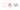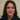# What is spread syntax in ES6 and how to use it?

Continuing our explanation for ES6 new features, we will review the spread operator. What is the magic spread syntax in ES6? The spread syntax is simply three dots: `…`

``` It allows an iterable to expand in places where 0 or more arguments likeIndefinite number of arguments (function calls) or  elements (array literals) or key-value pairs (object literals) are expected. Let us have a look at some of its usages to get a better idea: Calling functions without Apply To call a function with array argument we needed to use apply and use this syntax: function doSomething (x, y, z) { } var args = [0, 1, 2]; // Call the function, passing args doSomething.apply(null, args); This old problem has been solved using the spread operator. So we can now omit apply and use the spread syntax to call the function like this: <span class="token punctuation">doSomething</span><span class="token punctuation">(...</span>args<span class="token punctuation">)</span><span class="token punctuation">;</span> It is that simple and fun! Copying arrays To copy an array we pass a reference to the second one so they will have same values: var arr = ['a', 'b', 'c']; var arr2 = arr; console.log(arr2); </div> </div> </div> </div> </div> </div> </div> </div> <div> <div class="queriable-output-wrapper" data-displayed-checksum="8182c6308fc7af0093e78351b885deaeea9e2aba" data-input-checksum="checksum-8182c6308fc7af0093e78351b885deaeea9e2aba"> <div class="embedStyle_1ki8n3b object-description"> <div class="value_142hmgp-o_O-monospace_5jrb82 flexBox"> <p> <span class="normal_t5s8ur"><span class="fixme-test-wrapper">output: [<span class="type-string">"a"</span>, <span class="type-string">"b"</span>, <span class="type-string">"c"</span>]</span></span> </p> <p> As expected. And if we add an item to the second array like this: </p> <p> arr2.push('d'); </p> <p> console.log(arr); </p> <p> output: ['a', 'b', 'c', 'd'] </p> <p> Not expected. We want this to be added only to array 2 so spread operator is here just in he right place: </p> <p> var arr = ['a', 'b', 'c']; </p> <p> var arr2 = [...arr]; // this is where the magic happens </p> <p> console.log(arr2); </p> <p> output: ['a', 'b', 'c'] </p> <p> Now we add the item: </p> <p> arr2.push('d'); </p> <p> console.log(arr); </p> <p> output: ['a', 'b', 'c'] </p> <p> And as expected, we get the right results. The first array hasn&#8217;t changed now. </p> <h2 id="fa14" class="graf graf--h4 graf-after--p"> Convet string to array </h2> <p> We could use spread syntax to convert string to an array: </p> <p> var str = "hello"; </p> <p> var chars = [...str]; </p> <p> console.log(chars); </p> <p> output: [<span class="type-string">"h"</span>, <span class="type-string">"e"</span>, <span class="type-string">"l"</span>, <span class="type-string">"l"</span>, <span class="type-string">"o"</span>] </p> <h2> Combine arrays </h2> <p> We used to combine arrays or insert an array into another as shown in this example: </p> <p> var mid = [3, 4]; </p> <p> var arr = [1, 2, mid, 5, 6]; </p> <p> console.log(arr); </p> <p> output: [1, 2, [3, 4], 5, 6] </p> <p> But this is not quiet satisfying but with spread operator you could get the expected result: </p> <p> <span style="font-family: georgia, palatino, serif;">var mid = [3, 4];</span> </p> <p> <span style="font-family: georgia, palatino, serif;">var arr = [1, 2, ...mid, 5, 6];</span> </p> <p> <span style="font-family: georgia, palatino, serif;">console.log(arr);</span> </p> <p id="2b6d" class="graf graf--pre graf-after--p"> <span style="font-family: georgia, palatino, serif;">output: [1, 2, 3, 4, 5, 6]</span> </p> <p class="graf graf--pre graf-after--p"> As we expected. Also there are other ways of using spread operator to combine arrays: </p> <p class=" language-js">  // Adds arr2 items to end of array </p> <p> arr1.push(...arr2) </p> <p class=" language-js"> //Adds arr2 items to the beginning of array </p> <p class=" language-js"> arr1.unshift(...arr2)  </p> <h2> Using spread syntax in Math functions </h2> <p> Spread operator &#8220;spreads&#8221; an array into different arguments, so any function where spread is used as the argument can be used by functions that can accept any number of arguments. <code class="markup--code markup--p-code">Math.max() returns the largest of zero or more numbers.  </p> <p class=" language-js"> <span class="token keyword">let</span> numbers <span class="token operator">=</span> <span class="token punctuation">[</span><span class="token number">9</span><span class="token punctuation">,</span> <span class="token number">4</span><span class="token punctuation">,</span> <span class="token number">7</span><span class="token punctuation">,</span> <span class="token number">1</span><span class="token punctuation">]</span><span class="token punctuation">;</span> </p> <p class=" language-js"> Math<span class="token punctuation">.</span><span class="token function">max</span><span class="token punctuation">(</span><span class="token operator">...</span>numbers<span class="token punctuation">)</span><span class="token punctuation">;</span> <span class="token comment" spellcheck="true">// outputs: 9</span> </p> <p> The Math object&#8217;s set of functions are a perfect example of the spread operator as the only argument to a function. </p> <h2> Destructing with spread operator </h2> <p> With spread operator we can destruct an array using <a href="https://developer.mozilla.org/en-US/docs/Web/JavaScript/Reference/Functions/rest_parameters" target="_blank" rel="noopener noreferrer">rest</a> and spread syntax at the same time: </p> <p> let { x, y, ...z } = { x: 1, y: 2, a: 3, b: 4 }; </p> <p> console.log(x); // 1 </p> <p> console.log(y); // 2 </p> <p> console.log(z); // { a: 3, b: 4 }  </p> <p> The rest of the properties are assigned to the variable after the spread operator! </p> <p> With spread syntax in our hands, Javascript is more fun than ever! </p> </div> </div> </div> </div>```
`© 2020This site is created and maintined by Azadeh Faramarzi , A passionate developer, sport and code lover who loves to share and write when she has some time. `